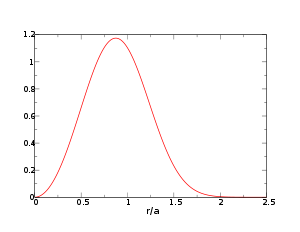# Mean inter-particle distance

Mean inter-particle distance

Mean inter-particle distance (or mean inter-particle separation) is the mean distance between microscopic particles (usually atoms or molecules) in a macroscopic body.

## Ambiguity

From the very general considerations, the mean inter-particle distance is proportional to the size of the per-particle volume 1 / n, i.e.,$\langle r \rangle \sim 1/n^{1/3},$

where n = N / V is the particle density. However, barring a few simple cases such as the ideal gas model, precise calculations of the proportionality factor are impossible analytically. Therefore, approximate expressions are often used. One such an estimation is the Wigner-Seitz radius$\left( \frac{3}{4 \pi n} \right)^{1/3},$

which corresponds to the radius of a sphere having per-particle volume 1 / n. Another popular definition is

1 / n1 / 3,

corresponding to the length of the edge of the cube with the per-particle volume 1 / n. Evidently, the two definitions differ by a factor of$\approx 1.61$, thus one has to exercise care if an article fails to define the parameter exactly. On the other hand, it is often used in qualitative statements where such a numeric factor is either irrelevant or plays an insignificant role, e.g.,

• "a potential energy ... is proportional to some power n of the inter-particle distance r" (Virial theorem)
• "the inter-particle distance is much larger than the thermal de Broglie wavelength" (Kinetic theory)

## Ideal gas

### Nearest neighbor distributionPDF of the NN distances in an ideal gas.

We want to calculate probability distribution function of distance to the nearest neighbor (NN) particle. (The problem was first considered by Paul Hertz; for a modern derivation see, e.g.,.) Let us assume N particles inside a sphere having volume V, so that n = N / V. Note that since the particles in the ideal gas are non-interacting, the probability to find a particle at a certain distance from another particle is the same as probability to find a particle at the same distance from any other point; we shall use the center of the sphere.

An NN particle at distance r means exactly one of the N particles resides at that distance while the rest N − 1 particles are at larger distances, i.e., they are somewhere outside the sphere with radius r.

The probability to find a particle at the distance from the origin between r and r + dr is$N \frac{4 \pi r^2 dr}{V}$, while the probability to find a particle outside that sphere is$1 - \frac{4\pi}{3}r^3/V$. The sought-for expression is then$P_N(r)dr = 4 \pi r^2 dr\frac{N}{V}\left(1 - \frac{4\pi}{3}r^3/V \right)^{N - 1} = \frac{3}{a}\left(\frac{r}{a}\right)^2\left(1 - \left(\frac{r}{a}\right)^3 \frac{1}{N} \right)^{N - 1}\,,$

where we substituted$a = \left( \frac{3}{4 \pi n} \right)^{1/3}.$

Finally, taking the$N \rightarrow \infty$ limit and using$\lim_{x \rightarrow \infty}\left(1 + \frac{1}{x}\right)^x = e$, we obtain$P(r) = \frac{3}{a}\left(\frac{r}{a}\right)^2 e^{-(r/a)^3}\,.$

One can immediately check that$\int_{0}^{\infty}P(r)dr = 1\,.$

The distribution peaks at$r_{\text{peak}} = \left(\frac{2}{3}\right)^{1/3} a \approx 0.874 a\,.$

### Mean distance and higher NN distribution moments$\langle r^k \rangle = \int_{0}^{\infty}P(r) r^k dr = 3 a^k\int_{0}^{\infty}x^{k+2}e^{-x^3}dx\,,$

or, using the t = x3 substitution,$\langle r^k \rangle = a^k \int_{0}^{\infty}t^{k/3}e^{-t}dt = a^k \Gamma(1 + \frac{k}{3})\,,$

where Γ is the gamma function. Thus,$\langle r^k \rangle = a^k \Gamma(1 + \frac{k}{3})\,.$

In particular,$\langle r \rangle = a \Gamma(\frac{4}{3}) = \frac{a}{3} \Gamma(\frac{1}{3}) \approx 0.893 a\,.$

Wikimedia Foundation. 2010.

### Look at other dictionaries:

• Dissipative particle dynamics — (DPD) is a stochastic simulation technique for simulating the dynamic and rheological properties of simple and complex fluids. It was initially devised by Hoogerbrugge and Koelman  to avoid the lattice artifacts of the so called lattice gas …   Wikipedia

• Kinetic theory — [ temperature of an ideal monatomic gas is a measure related to the average kinetic energy of its atoms as they move. In this animation, the size of helium atoms relative to their spacing is shown to scale under 1950 atmospheres of pressure.… …   Wikipedia

• Virial theorem — In mechanics, the virial theorem provides a general equation relating the average over time of the total kinetic energy, , of a stable system consisting of N particles, bound by potential forces, with that of the total potential energy, , where… …   Wikipedia

• Van der Waals equation — The van der Waals equation is an equation of state for a fluid composed of particles that have a non zero size and a pairwise attractive inter particle force (such as the van der Waals force.) It was derived by Johannes Diderik van der Waals in… …   Wikipedia

• Lennard-Jones potential — Computational physics Numerical analysis  …   Wikipedia

• Mathematics and Physical Sciences — ▪ 2003 Introduction Mathematics       Mathematics in 2002 was marked by two discoveries in number theory. The first may have practical implications; the second satisfied a 150 year old curiosity.       Computer scientist Manindra Agrawal of the… …   Universalium

• river — river1 riverless, adj. riverlike, adj. /riv euhr/, n. 1. a natural stream of water of fairly large size flowing in a definite course or channel or series of diverging and converging channels. 2. a similar stream of something other than water: a… …   Universalium

• Thermodynamic temperature — is the absolute measure of temperature and is one of the principal parameters of thermodynamics. Thermodynamic temperature is an “absolute” scale because it is the measure of the fundamental property underlying temperature: its null or zero point …   Wikipedia

• List of statistics topics — Please add any Wikipedia articles related to statistics that are not already on this list.The Related changes link in the margin of this page (below search) leads to a list of the most recent changes to the articles listed below. To see the most… …   Wikipedia

• international relations — a branch of political science dealing with the relations between nations. [1970 75] * * * Study of the relations of states with each other and with international organizations and certain subnational entities (e.g., bureaucracies and political… …   Universalium